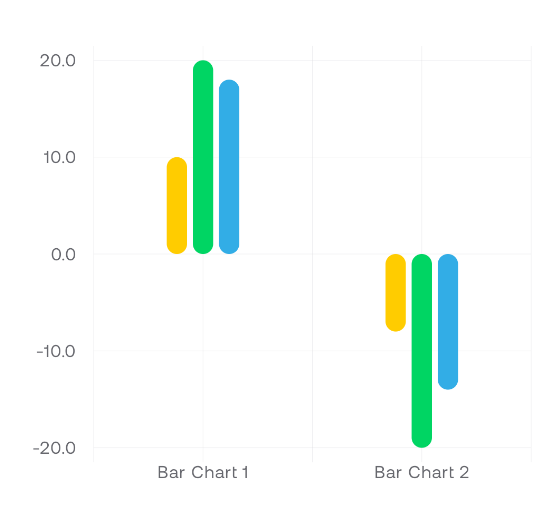Before using component the BarChartData has to be prepared:

```val barChartData = BarChartData(
categories = listOf(
BarChartCategory(
name = "Bar Chart 1",
entries = listOf(
BarChartEntry(
x = "primary",
y = 17f,
color = Color.Yellow,
),
BarChartEntry(
x = "secondary",
y = 30f,
color = Color.Red,
),
)
),
BarChartCategory(
name = "Bar Chart 2",
entries = listOf(
BarChartEntry(
x = "primary",
y = -5f,
color = Color.Yellow,
),
BarChartEntry(
x = "secondary",
y = -24f,
color = Color.Red,
),
)
),
)
)```

```BarChart(
data = barChartData,
config = BarChartConfig(
thickness = 14.dp,
),
modifier = Modifier.height(500.dp),
animation = ChartAnimation.Sequenced(),
)```

There is another component called `BarChartWithLegend`. It renders bar chart with legend.

## BubbleChart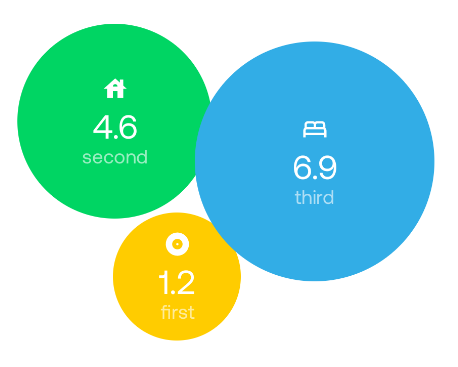Before using component the list of Bubble has to be prepared:

```val bubbles = listOf(
Bubble(
name = "first",
value = 1.2f,
icon = Icons.Default.Album,
color = Color.Yellow
),
Bubble(
name = "second",
value = 4.6f,
icon = Icons.Default.House,
color = Color.Green
),
Bubble(
name = "third",
value = 6.9f,
icon = Icons.Default.Bed,
color = Color.Blue
),
)```

```BubbleChart(
bubbles = bubbles,
modifier = Modifier.size(300.dp),
animation = ChartAnimation.Sequenced(),
)```

# Dial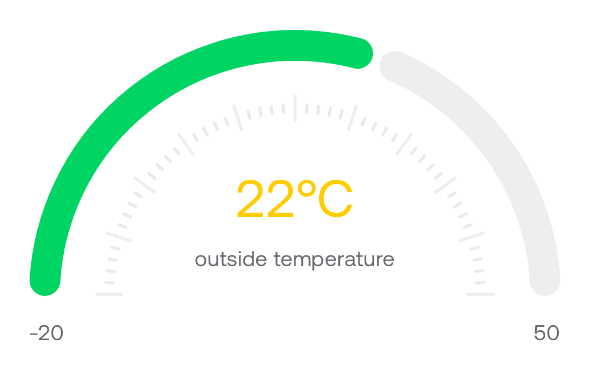```Dial(
value = 22,
minValue = -20,
maxValue = 50,
modifier = Modifier.fillMaxWidth(),
animation = ChartAnimation.Simple {
spring(
dampingRatio = Spring.DampingRatioMediumBouncy,
stiffness = Spring.StiffnessLow
)
},
config = DialConfig(
thickness = 20.dp,
roundCorners = true,
),
mainLabel = {
Column(
horizontalAlignment = Alignment.CenterHorizontally
) {
Text(
text = "\$it°C",
style = MaterialTheme.typography.h4,
color = Color.Yellow
)
Text(
text = "outside temperature",
style = MaterialTheme.typography.body2,
)
}
}
)```

There is another component `ProcentageDial`. It accepts only one data argument `percentage` in [0-100] range.

# GasBottle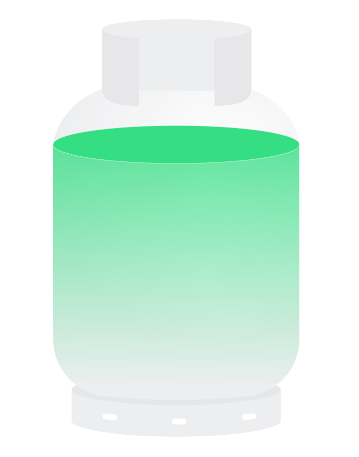```GasBottle(
percentage = 75f,
modifier = Modifier.size(width = 200.dp, height = 300.dp),
animation = ChartAnimation.Simple {
spring(
dampingRatio = Spring.DampingRatioMediumBouncy,
stiffness = Spring.StiffnessVeryLow
)
}
)```

# LineChart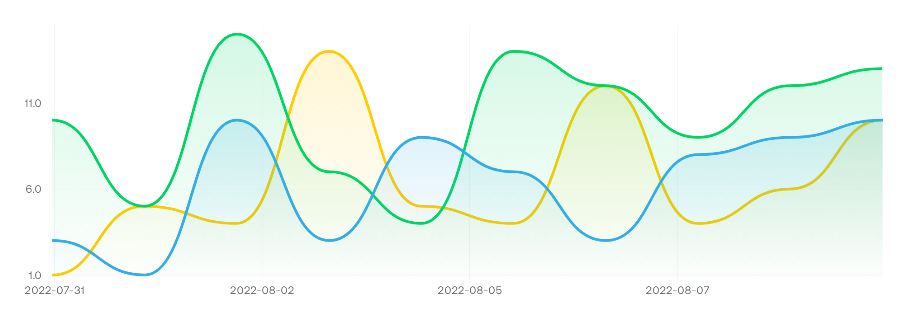Before using component the LineChartData has to be prepared:

```val lineData = remember {
LineChartData(
series = (1..3).map {
LineChartSeries(
dataName = "data \$it",
lineColor = listOf(
Color.Yellow,
Color.Red,
Color.Blue,
)[it - 1],
listOfPoints = (1..10).map { point ->
LineChartPoint(
x = DateTime.now().minus(TimeSpan(point * 24 * 60 * 60 * 1000.0)).unixMillisLong,
y = (1..15).random().toFloat(),
)
}
)
},
)
}```

```LineChart(
lineChartData = lineData,
modifier = Modifier.height(300.dp),
xAxisLabel = {
Text(
fontSize = 12.sp,
text = DateTime.fromUnix(it as Long).format("yyyy-MM-dd"),
textAlign = TextAlign.Center
)
},
Text(
text = DateTime.fromUnix(it as Long).format("yyyy-MM-dd"),
style = MaterialTheme.typography.overline
)
},
animation = ChartAnimation.Sequenced()
)```

# PieChart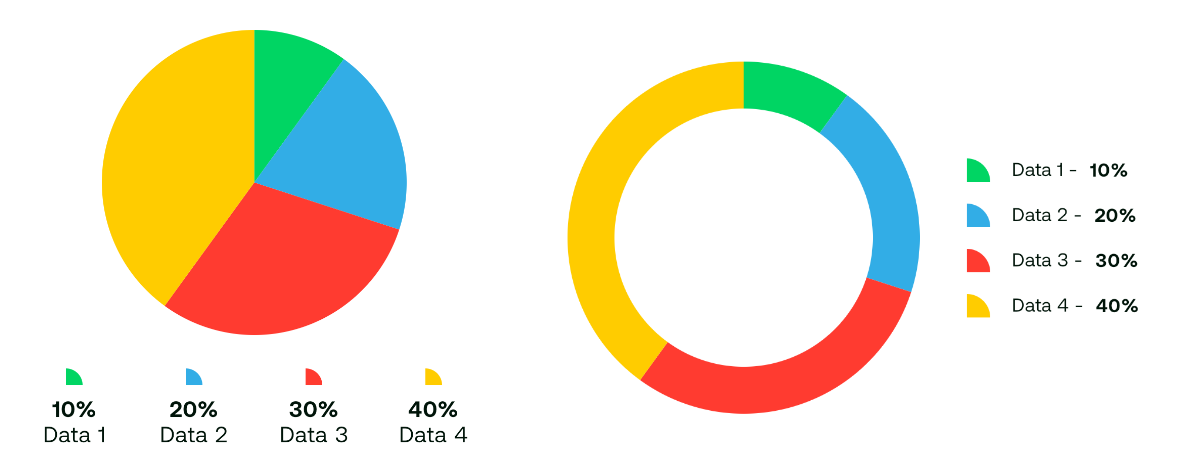Before using component the list of PieChartData has to be prepared:

```val data = listOf(
PieChartData(
name = "Data 1",
value = 10.0,
color = Color.Yellow,
),
PieChartData(
name = "Data 2",
value = 20.0,
color = Color.Green,
),
PieChartData(
name = "Data 3",
value = 30.0,
color = Color.Blue,
),
PieChartData(
name = "Data 4",
value = 40.0,
color = Color.Red,
)
)```

```PieChart(
data = data,
modifier = Modifier.size(300.dp),
config = PieChartConfig(
thickness = 40.dp
),
)```

By default the thickness is `Dp.Infinity`, it means the chart will be fully filled.

# Theming

The easiest way to set the same colors for all charts is to provide `ChartColors` in the app theme.

```private val chartColors = ChartColors(
primary = Color.Green,
grid = Color.LightGray,
surface = Color.White,
fullGasBottle = Color.Green,
emptyGasBottle = Color.Red,
overlayLine = Color.Magenta
)

@Composable
fun AppTheme(
darkTheme: Boolean = isSystemInDarkTheme(),
content: @Composable () -> Unit,
) {
CompositionLocalProvider(
// ...
LocalChartColors provides chartColors,
) {
MaterialTheme(
// ...
content = content,
)
}
}
```

There is also default ChartColors provided by the library. It uses the default color set from `MaterialTheme`.

`LocalChartColors provides ChartDefaults.chartColors()`

Each chart has its own color set which can be used like:

```BarChart(
data = barChartData,
colors = BarChartColors(grid = Color.LightGray)
)```

Also there is possibility to use ChartColors inside the specific chart:

```BarChart(
data = barChartData,
colors = ChartColors(...).barChartColors,
)```

# Contributing

Feel free to create a PR.